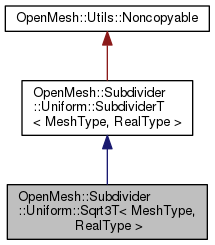OpenMesh
OpenMesh::Subdivider::Uniform::Sqrt3T< MeshType, RealType > Class Template Reference

Uniform Sqrt3 subdivision algorithm More...

`#include <OpenMesh/Tools/Subdivider/Uniform/Sqrt3T.hh>`

Inheritance diagram for OpenMesh::Subdivider::Uniform::Sqrt3T< MeshType, RealType >:[legend]
Collaboration diagram for OpenMesh::Subdivider::Uniform::Sqrt3T< MeshType, RealType >:[legend]

## Public Types

typedef RealType real_t

typedef MeshType mesh_t

typedef SubdividerT< mesh_t, real_t > parent_t

typedef std::pair< real_t, real_t > weight_t

typedef std::vector< std::pair< real_t, real_t > > weights_tPublic Types inherited from OpenMesh::Subdivider::Uniform::SubdividerT< MeshType, RealType >
typedef MeshType mesh_t

typedef RealType real_t

## Public Member Functions

Sqrt3T (MeshType &_m)

const char * name () const
Return name of subdivision algorithm.

void init_weights (size_t _max_valence=50)
Pre-compute weights.Public Member Functions inherited from OpenMesh::Subdivider::Uniform::SubdividerT< MeshType, RealType >
virtual ~SubdividerT ()
Descructor (calls detach())

SubdividerT (void)
Constructor to be used with interface 2. More...

SubdividerT (MeshType &_m)
Constructor to be used with interface 1 (calls attach()) More...

bool operator() (MeshType &_m, size_t _n, const bool _update_points=true)
Subdivide the mesh `_m` `_n` times. More...

bool attach (MeshType &_m)
Attach mesh `_m` to self. More...

bool operator() (size_t _n, const bool _update_points=true)
Subdivide the attached `_n` times. More...

void detach (void)
Detach an eventually attached mesh. More...

## Protected Member Functions

bool prepare (MeshType &_m)

bool cleanup (MeshType &_m)
Cleanup mesh after usage, e.g. remove added properties.

bool subdivide (MeshType &_m, size_t _n, const bool _update_points=true)
Subdivide mesh `_m` `_n` times. More...

## Detailed Description

### template<typename MeshType, typename RealType = float> class OpenMesh::Subdivider::Uniform::Sqrt3T< MeshType, RealType >

Uniform Sqrt3 subdivision algorithm

Implementation as described in

L. Kobbelt, "Sqrt(3) subdivision", Proceedings of SIGGRAPH 2000.

## Member Function Documentation

template<typename MeshType , typename RealType = float>
 bool OpenMesh::Subdivider::Uniform::Sqrt3T< MeshType, RealType >::subdivide ( MeshType & _m, size_t _n, const bool _update_points = `true` )
inlineprotectedvirtual

Subdivide mesh `_m` `_n` times.

TODO:Implement fixed positions

The documentation for this class was generated from the following file:
• OpenMesh/Tools/Subdivider/Uniform/Sqrt3T.hh

Project OpenMesh, ©  Computer Graphics Group, RWTH Aachen. Documentation generated using doxygen .